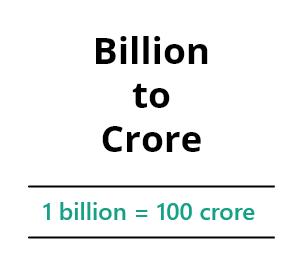# Billion to Crore/Crore to Billion Converter

To convert numbers from billion to crore or crore to billion, enter the value and hit calculate button

Give Us Feedback

## Billion to Crore Converter

The Billion to crore/crore to billion converter (it can be used both ways) is an online calculator that converts values from one numbering system to another.

Other than providing the calculating result for the input value, it gives other conversions such as billion to lakhs, million, and trillions.

## What is a billion to crore conversion?

Billion is the word used to represent a value containing 9 zeros or 10 digits in the international numbering system. On the other hand, the word crore accounts for a value containing 7 zeros or 8 digits in the Indian numbering system.

The conversion is done so that people using either system can clearly understand the volume of value or amount.### Billion:

The numerical representation of billion is 1,000,000,000. It is a place value used internationally except for a few countries.

### Crore:

Crore is written in numbers as 1,00,00,000. It is most commonly used in South Asian countries like Pakistan, Bangladesh, India, and Sri Lanka.

In billion to crore conversion, you are not only representing the numbers in terms of a different place value but also a different numbering system.

One billion(109) equates to 1000 million (106). This conversion is the place value conversion. On the other hand, one billion is also equal to 100 crores (107). Here, you are jumping from one numbering system to another.

## How to convert billions to crores?

For this, use a crore to billion converter. But if you wish to perform the conversion manually, then keep in mind the following two points.

• One billion is equal to 100 crores. Multiply the values in billions with 100 to know how much they make in crores.
• One crore is equal to 0.01 billion. Divide the crores by 100 to convert them into billions.

Example:

How many crores are in 78 billion?

Solution:

1 billion = 100 crore
78 billions = 78 x 100 crores
= 7800 crores

## Conversion tables:

### Billion to core:

 1 Billion 100 Crore 5 Billion 500 Crore 10 Billion 1000 Crore 20 Billion 2000 Crore 100 Billion 10,000 Crore 10,000 Billion 10,00,000 Crore 65 Billion 6500 Crore 2,356 Billion 2,35,600 Crore 12.35 Billion 1235 Crore 2/5 Billion 40 Crore

### Crore to billion:

 1 Crore 0.01 Billion 5 Crore 0.05 Billion 10 Crore 0.1 Billion 100 Crore 1 Billion 200 Crore 2 Billion 500 Crore 5 Billion 1000 Crore 10 Billion 10,00,000 Crore 10,000 Billion 111.7 Crore 1.117 Billion 2598 Crore 25.98 Billion

## FAQs

Are 1000 crores a billion?

No, 1000 crores to billion are 10 billion. One billion is equal to 100 (hundred) crores.

Convert 400 billion to crores.

Four hundred billion equals forty thousand crores. As 1 billion is equal to 100 (hundred)  crores so, 400 billion = 40,000 crores.

How much means 1 billion?

In general, 1 billion is mathematically written as

1 billion = 1,000,000,000

To write such a larger term mathematician denotes it by one billion.

How many crores makes 300 billion?

As we know that 1 billion is equal to one hundred (100) crores. Similarly,

300 billion = 300 x 100 crore

300 billion = 30,000 crores

Hence, 30,000 crores make 300 billion.